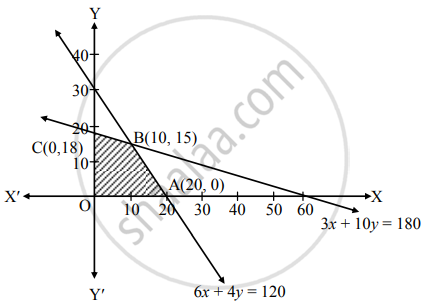# Solve the following problem : A company manufactures bicyles and tricycles, each of which must be processed through two machines A and B Maximum availability of machine A and B is respectively 120 a - Mathematics

Graph
Sum

Solve the following problem :

A company manufactures bicyles and tricycles, each of which must be processed through two machines A and B Maximum availability of machine A and B is respectively 120 and 180 hours. Manufacturing a bicycle requires 6 hours on machine A and 3 hours on machine B. Manufacturing a tricycle requires 4 hours on machine A and 10 hours on machine B. If profits are ₹ 180 for a bicycle and ₹ 220 on a tricycle, determine the number of bicycles and tricycles that should be manufacturing in order to maximize the profit.

#### Solution

Let x number of bicycles and y number of tricycles be manufactured by the company.
∴ Total profit Z = 180x + 220y
This is the objective function to be maximized.
The given information can be tabulated as shown below:

 Bicycles (x) Tricycles (y) Maximum availability of time (hrs) Machine A 6 4 120 Machine B 3 10 180

∴ The constraints are 6x + 4y ≤ 120, 3x + 10y ≤ 180, x ≥ 0, y ≥ 0
∴ Given problem can be formulated as
Maximize Z = 180x + 220y
Subject to, 6x + 4y ≤ 120, 3x + 10y ≤ 180 , x ≥ 0, y ≥ 0.
To draw the feasible region, construct the table as follows:

 Inequality 6x + 4y ≤ 120 3x + 10y ≤ 180 Corresponding equation (of line) 6x + 4y = 120 3x + 10y = 180 Intersection of line with X-axis (20, 0) (60, 0) Intersection of line with Y-axis (0, 30) (0, 18) Region Origin side Origin side

Shaded portion OABC is the feasible region,
whose vertices are O ≡ (0, 0), A ≡ (20, 0), B and C ≡ (0, 18)
B is the point of intersection of the lines 3x + 10y = 180 and 6x + 4y = 120.
Solving the above equations, we get
B ≡ (10, 15)
Here the objective function is,
Z = 180x + 220y
∴ Z at O(0, 0) = 180(0) + 220(0) = 0
Z at A(20, 0) = 180(20) + 220(0) = 3600
Z at B(10, 15) = 180(10) + 220(15) = 5100
Z at C(0, 18) = 180(0) + 220(18) = 3960
∴ Z has maximum value 5100 at B(10, 15)
∴ Z is maximum when x = 10, y = 15Thus, the company should manufacture 10 bicycles and 15 tricycles to gain maximum profit of ₹ 5100.

Concept: Mathematical Formulation of Linear Programming Problem
Is there an error in this question or solution?

#### APPEARS IN

Balbharati Mathematics and Statistics 2 (Commerce) 12th Standard HSC Maharashtra State Board
Chapter 6 Linear Programming
Miscellaneous Exercise 6 | Q 4.09 | Page 104